Next: 5.2 Modeling Approaches Up: 5. Impact-Ionization Generation Previous: 5. Impact-Ionization Generation

Subsections

# 5.1 Basics of Impact-Ionization

Impact-ionization is a three-particle generation process. Carriers that gain high energies while traveling through high field regions undergo scattering events with bonded electrons in the valence band. The excess energy is transferred to this electron which is lifted into the conduction band creating a new electron-hole pair. This secondary electron-hole pair can also have a rather high energy. In this case the avalanche effect is triggered and the carrier density increases heavily. This effect is sketched for pure electron induced generation in Fig. 5.1.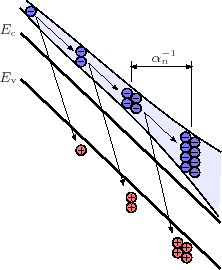The device behavior is heavily affected by impact-ionization. In MOS devices, the peak of generation is commonly observed in the channel near the drain area. The minority carriers contribute to the drain current, while the majority carriers are attracted and collected by the bulk electrode. A common measure for the occurrence of impact-ionization in MOS devices is, therefore, the bulk current. For reverse-biased pn-junctions, for example, the avalanche breakdown usually defines the maximum blocking voltage. To overcome this, doping profiles are specially graded to decrease the maximum fields for a given voltage. Unfortunately, this often has a detrimental impact on the forward behavior. Therefore, application specific trade offs between forward and reverse behaviors have to be found (see also Section 2.2.2). Moreover, also forward biased pn-junctions suffer due to impact-ionization. The additionally generated carriers increase the forward currents in transistors. In the worst-case, gate- (or base-control) can be lost. Increasing currents lead to massively growing heat generation and can finally lead to device failure. Especially bipolar transistors are vulnerable due to the positive temperature coefficient. Another effect which has to be considered in the device design are majority carrier current flows initiated by strong generation which can trigger parasitic devices, thereby leading to unexpected device behavior. Since impact-ionization has such a strong influence on the device behavior, it is compulsory to include proper models into device simulation tools.

## 5.1.1 Ionization Rate

In the drift-diffusion model, the impact-ionization rate is usually expressed using the impact-ionization coefficients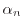and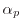. These two coefficients describe the number of electron-hole pairs generated per unit distance traveled  by a solitary carrier between two collisions. Considering only electrons generated by electrons, one can write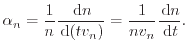(5.1)

Here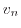is the electron velocity and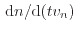therefore describes the generated carriers per distance.is the electron concentration andthe electron generation rate. Accounting also for the generated holes and for the holes that contribute to the impact-ionization multiplication, the generation rate can be written as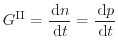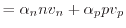(5.2)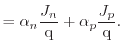(5.3)

The coefficientsandin bulk silicon, as shown in Fig. 5.2, are strongly field dependent. A typical model is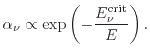(5.4)

A discussion on modeling of the impact-ionization coefficients in device simulation tools will be given in Section 5.2.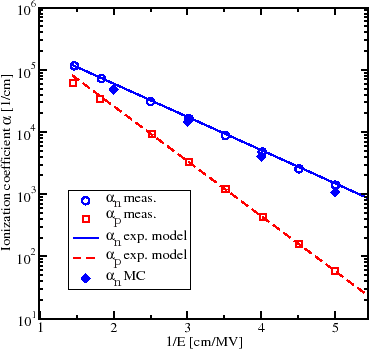## 5.1.2 Ionization Integral

To describe the impact-ionization caused avalanche breakdown, for example, in reverse-biased junctions, a macroscopic formalism called ionization integral is introduced. This quantity is based on the impact-ionization coefficients. It considers carriers which enter the depletion region and are then accelerated by the electric field. The carriers gain enough energy to generate new electron-hole pairs by impact-ionization. If these newly generated carriers have high enough energy they can launch a secondary branch of impact-ionization. Therefore, the entire process can be cascaded, leading to avalanche carrier multiplication. This results in high currents and eventually leads to breakdown.

For a one-dimensional consideration, a depletion region with width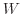is assumed. An initial hole current density at the position of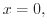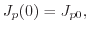is considered to trigger the avalanche. The density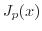steadily increases throughout the depletion region due to the holes generated by impact-ionization as shown in Fig. 5.3(a).

The simultaneously generated electrons are accelerated in the opposite direction resulting in an electron current density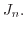In steady-state, the total current density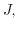defined as(5.5)

must be constant along the device. At the end of the depletion region, i.e. at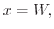the hole current reaches its maximum which is described by applying the multiplication factor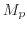as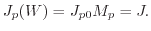(5.6)

Using (5.3) and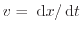the change of the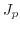passing through the depletion region is evaluated as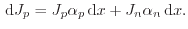(5.7)

Together with (5.5) one reformulates to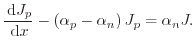(5.8)

Applying the stated boundary conditions, this differential equation can be solved, as shown in Appendix A, to yield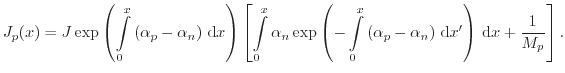(5.9)

At the positionthe hole current density becomes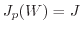and (5.9) evaluates to (see Appendix A)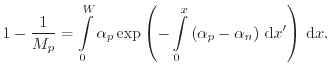(5.10)

The avalanche breakdown sets in if the multiplication factorapproaches infinity. The relation between depletion region width and the ionization coefficients at breakdown therefore reads(5.11)

The derivation for an initial electron current instead of an initial hole current leads to an equivalent result  and it therefore does not make a difference which carrier type triggers the avalanche breakdown. In the case shown in Fig. 5.3(b) the ionization coefficientsandhave been set equal to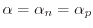and the ionization integral now reads(5.12)

Using the ionization integral the correlation between the width () of the depletion region and the ionization coefficientsandcan be derived at least for simplified structures and coefficient models. The current density is not required for modeling of the breakdown condition. Hence, for evaluation of the breakdown voltage one may solve the simplified Poisson equation by assuming constant quasi-Fermi levels in the depletion region.

Figure 5.3: Electron and hole current densities (a) and ionization coefficient, rate, and ionization integral (b) in a one dimensional simulation of a reverse-biased diode operated at breakdown. The abrupt pn-junction at the position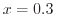µm is n-doped (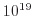cm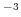) in the right and p-doped (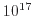cm) in the left side. The ionization coefficients are set equal (). Equal coefficients and constant total current lead to the overlapping shape of the ionization coefficient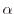and ionization rate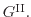The ionization integral (5.12) at the bottom of (b) equals 1 at the end of the depletion zone.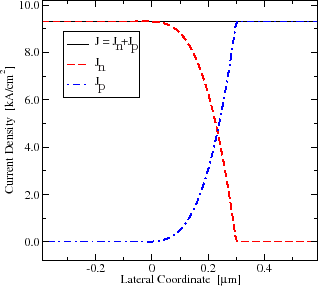(a)(b)

Obtaining the numerical solution using this approach saves a lot of computational power. However, due to severe simplifications required to compute this integral, this method is not relevant for real devices. An important application field of the ionization integral is the measurement of the ionization rate. The latter quantity is not achievable in direct measurements, and thus the ionization integral can be used to conclude on the ionization rate using breakdown conditions.Next: 5.2 Modeling Approaches Up: 5. Impact-Ionization Generation Previous: 5. Impact-Ionization Generation

O. Triebl: Reliability Issues in High-Voltage Semiconductor Devices